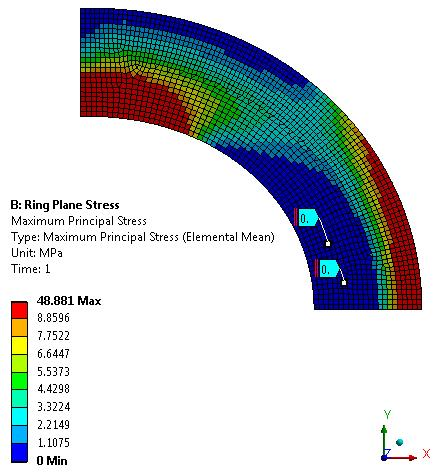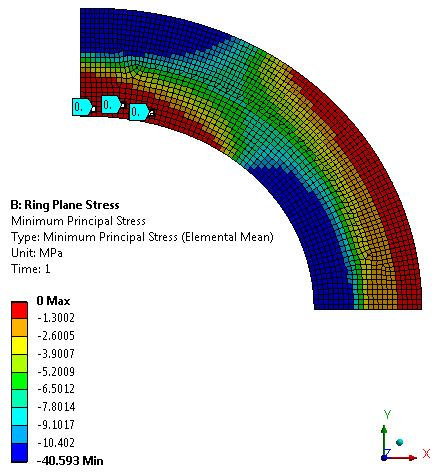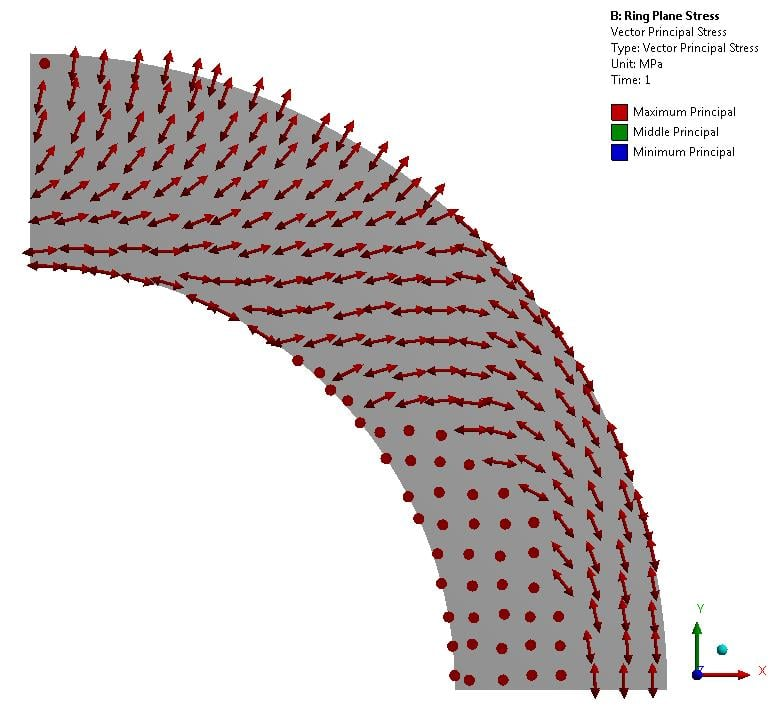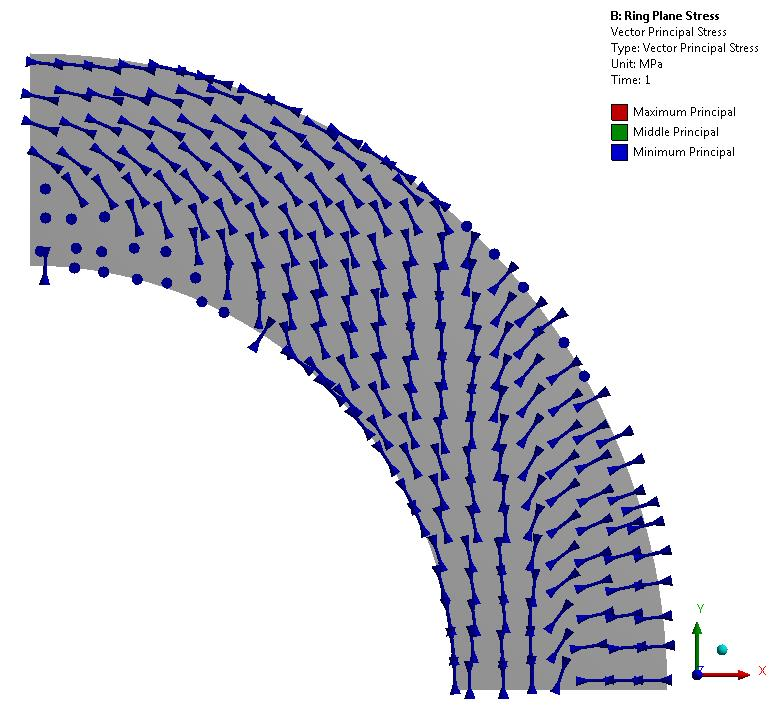## General Mechanical

•abhi939
Subscriber

Hello All,

I have a quadrant of a ring (with symmetry boundary conditions) that is subjected to an inplane force. Being a plane stress 2D problem, I was expecting a principle stress of zero magnitude in the out-of-plane direction. However, none of the Principle Stresses (Max, Middle, Min, S1, S2, S3) is zero. How is this possible?

I have attached my model as well. Any help would be appreciated.

•abhi939
Subscriber
•peteroznewman
Subscriber

I made one small edit to your model, I changed the force from a vertex to the vertical line. This removed the stress singularity.

You do have elements with zero principal stress values. Here is Max. Principal Stress.Below is Min Principal Stress.In what way does this not meet your expectations?

Regards,

Peter

•abhi939
Subscriber

My concern is that shouldn't all the elements have zero stress in the out of plane direction (sigma z-axis) since it is a plane stress condition? And shouldn't this axis be the principal stress direction and thus making one of the principal stress zero everywhere (and not just a part)?

PS: If you evaluate Sz (userdefined result), it is clearly shown to be zero everywhere. Why does it not correspond to the one of the principal stress?

Also can you send me your model? I want to see the edit you made and learn. Thanks a lot.

•peteroznewman
Subscriber

Your first sentence asks why all the elements don't have zero Normal stress in the Z direction, then your PS says that all the elements clearly do have a zero Normal stress in the Z direction. Haven't you answered your own question?

You can plot the principal stress direction. Below is the Max Principal Stress Vector Plot.  The dots are where the Max. Principal Stress is zero.You can also plot the Min Principal Stress direction, which is orthogonal to the Max Principal Stress vector.Regards,

Peter

Attached is an ANSYS 19.1 archive.

Guidelines for Posting

•abhi939
Subscriber

Hey there! Thank you for all the replies.

I think I didn't convey my question properly.

So as a plane stress problem this analysis should have had a principle stress direction out of plane. The magnitude of this principle stress should be zero ( since it is a 2D Plane Stress problem).

Moving to ansys analysis, I evaluated all the principal stresses (Max, Min, Middle) from the predefined stress results (solution--> insert--> stress--> Max, Min, Middle). Now atleast one of this had to be zero at every element! But none do.

Now the PS (post script) part that i said in previous reply. I checked through ansys documentation and found Sz to give stress in z-axis direction. So I evaluated that using userdefined result and found it to be zero everywhere which is what I expected. But this Sz should have been one of the principal stresses that I evaluated in the previous paragraph which, according to ansys, is clearly not the case. One of the principle stress plot should have matched Sz plot (=0 at all points)

•peteroznewman
Subscriber

When I plot the principal stresses, I am interested in the Max (tensile) and the Min (compressive) principal stress. I don't care about the Mid principal stress.

•abhi939
Subscriber

You are right, but to check the validity of model had a look at all of the principal stresses. How do I believe the results from Ansys if one of the principal stress is not what it should be from theory? If one of them is wrong so can the rest.

Also I did the same problem on ABAQUS and it showed the expected results with one principle stress zero everywhere.

•Anna12
Subscriber

Hello,

I have a similar problem at the moment. I investigate a 2D cantilever model and want to plot the eigenvectors via Matlab, so the vector principals file includes rotation angle sequence ThetaXY, ThetaYZ, and ThetaZX (around z, x and y-axis) and at this point I can't understand why there is a rotation around all three axis. Maybe you can say your opinion.

You also write about the evaluation of Sz by using an user defiened result. Which expression did you use there?

Thank you!

Regards

Anna12

•peteroznewman
Subscriber
Hello Anna
Please copy your content and paste into a New Discussion. You can then delete the post above. It's better to have a new discussion because then you "own" the discussion and get notified of replies and you can decide when your question is answered. Also your question is a bit different from the original discussion question.

Regards, Peter